## Determine the quadrant in which the terminal side of the given angle lies. 1,165 I II

Question

Determine the quadrant in which the terminal side of the given angle lies.

1,165

I

II

IIII

IV

in progress 0
5 months 2021-08-09T06:04:54+00:00 2 Answers 5 views 0

A. I

Step-by-step explanation:

The sum of the angles in the four quadrants equals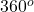.

Given an angle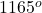, then;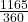= 3.236111….

So that,x 3 =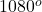Thus,=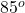We have;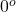<<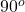Therefore, the terminal side would lie in the first quadrant. The correct option is A.